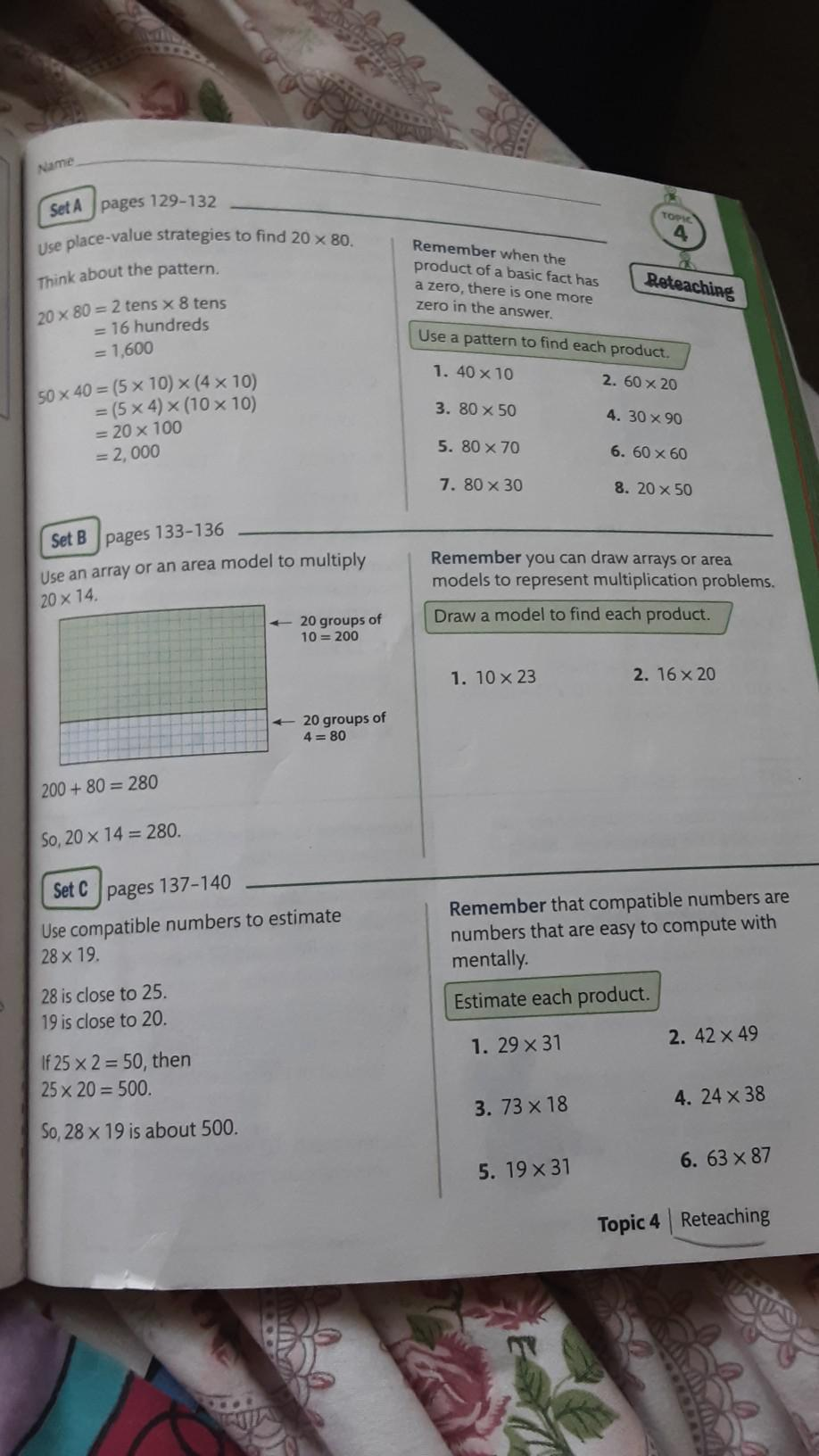Question:

# Name Set A pages 129-132 TOP 4 Use place-value strategies to find 20 X 80. Think about the pattern. Remember when the product ofName Set A pages 129-132 TOP 4 Use place-value strategies to find 20 X 80. Think about the pattern. Remember when the product of a basic fact has a zero, there is one more zero in the answer. Reteaching 20 x 80 = 2 tens x 8 tens = 16 hundreds = 1,600 Use a pattern to find each product. 1. 40 x 10 2. 60 x 20 3. 80 x 50 4. 30 x 90 50 x 40 = (5 x 10) (4 x 10) = (5 x 4) (10 x 10) = 20 x 100 = 2,000 5. 80 x 70 6. 60 x 60 7. 80 x 30 8. 20 x 50 Set B pages 133-136 Use an array or an area model to multiply Remember you can draw arrays or area models to represent multiplication problems. Draw a model to find each product. 20 x 14. + 20 groups of 10 = 200 1. 10 x 23 2. 16 x 20 + 20 groups of 4 = 80 200 + 80 = 280 So, 20 x 14 = 280. Set Cpages 137-140 Use compatible numbers to estimate 28 x 19. Remember that compatible numbers are numbers that are easy to compute with mentally Estimate each product. 28 is close to 25. 19 is close to 20. 1. 29 x 31 2. 42 x 49 If 25 x 2 = 50, then 25 x 20 = 500. 3. 73 x 18 4. 24 x 38 So, 28 x 19 is about 500. 6. 63 x 87 5. 19 x 31 Topic 4 Reteaching